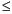Functions and CALL Routines

# PROBBNML Function

Returns the probability from a binomial distribution.
 Category: Probability See: CDF Function, PDF Function

## Syntax

 PROBBNML(p,n,m)

### Arguments

p

is a numeric probability of success parameter.

 RANGE: 0p1
n

is an integer number of independent Bernoulli trials parameter.

 RANGE: n > 0
m

is an integer number of successes random variable.

 RANGE: 0mn

The PROBBNML function returns the probability that an observation from a binomial distribution, with probability of success p, number of trials n, and number of successes m, is less than or equal to m. To compute the probability that an observation is equal to a given value m, compute the difference of two probabilities from the binomial distribution for m and m-1 successes.

SAS Statements Results
`x=probbnml(0.5,10,4);`
`0.376953125`

 Functions:Previous Page | Next Page | Top of Page